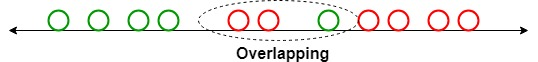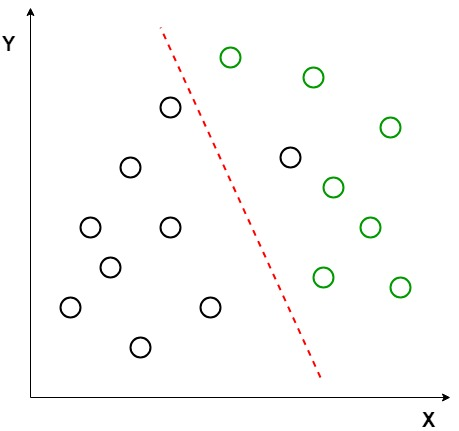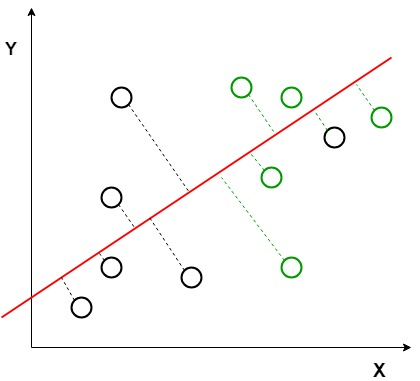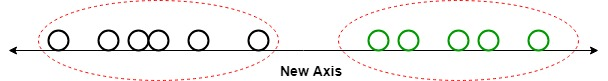# ML | Linear Discriminant Analysis

• Difficulty Level : Medium
• Last Updated : 24 Aug, 2022

Linear Discriminant Analysis or Normal Discriminant Analysis or Discriminant Function Analysis is a dimensionality reduction technique that is commonly used for supervised classification problems. It is used for modelling differences in groups i.e. separating two or more classes. It is used to project the features in higher dimension space into a lower dimension space.
For example, we have two classes and we need to separate them efficiently. Classes can have multiple features. Using only a single feature to classify them may result in some overlapping as shown in the below figure. So, we will keep on increasing the number of features for proper classification.Example:
Suppose we have two sets of data points belonging to two different classes that we want to classify. As shown in the given 2D graph, when the data points are plotted on the 2D plane, there’s no straight line that can separate the two classes of the data points completely. Hence, in this case, LDA (Linear Discriminant Analysis) is used which reduces the 2D graph into a 1D graph in order to maximize the separability between the two classes.Here, Linear Discriminant Analysis uses both the axes (X and Y) to create a new axis and projects data onto a new axis in a way to maximize the separation of the two categories and hence, reducing the 2D graph into a 1D graph.

Two criteria are used by LDA to create a new axis:

1. Maximize the distance between means of the two classes.
2. Minimize the variation within each class.In the above graph, it can be seen that a new axis (in red) is generated and plotted in the 2D graph such that it maximizes the distance between the means of the two classes and minimizes the variation within each class. In simple terms, this newly generated axis increases the separation between the data points of the two classes. After generating this new axis using the above-mentioned criteria, all the data points of the classes are plotted on this new axis and are shown in the figure given below.But Linear Discriminant Analysis fails when the mean of the distributions are shared, as it becomes impossible for LDA to find a new axis that makes both the classes linearly separable. In such cases, we use non-linear discriminant analysis.

Mathematics

Let’s suppose we have two classes and a d- dimensional samples such as x1, x2 … xn, where:

• n1 samples coming from the class (c1) and n2 coming from the class (c2).

If xi is the data point, then its projection on the line represented by unit vector v can be written as vTxi

Let’s consider u1 and u2 be the means of samples class c1 and c2 respectively before projection and u1hat denotes the mean of the samples of class after projection and it can be calculated by:Similarly,Now, In LDA we need to normalize |\widetilde{\mu_1} -\widetilde{\mu_2} |. Let y_i = v^{T}x_i  be the projected samples, then scatter for the samples of c1 is:Similarly:Now, we need to project our data on the line having direction v which maximizesFor maximizing the above equation we need to find a projection vector that maximizes the difference of means of reduces the scatters of both classes. Now, scatter matrix of s1 and s2 of classes c1 and c2 are:and s2After simplifying the above equation, we get:

Now, we define, scatter within the classes(sw) and scatter b/w the classes(sb):Now, we try to simplify the numerator part of J(v)Now, To maximize the above equation we need to calculate differentiation with respect to vHere, for the maximum value of J(v) we will use the value corresponding to the highest eigenvalue. This will provide us the best solution for LDA.

Extensions to LDA:

1. Quadratic Discriminant Analysis (QDA): Each class uses its own estimate of variance (or covariance when there are multiple input variables).
2. Flexible Discriminant Analysis (FDA): Where non-linear combinations of inputs are used such as splines.
3. Regularized Discriminant Analysis (RDA): Introduces regularization into the estimate of the variance (actually covariance), moderating the influence of different variables on LDA.

Implementation

• In this implementation, we will perform linear discriminant analysis using the Scikit-learn library on the Iris dataset.

## Python3

 # necessary importimport numpy as npimport pandas as pdimport matplotlib.pyplot as pltimport sklearnfrom sklearn.preprocessing import StandardScaler, LabelEncoderfrom sklearn.model_selection import train_test_splitfrom sklearn.discriminant_analysis import LinearDiscriminantAnalysisfrom sklearn.ensemble import RandomForestClassifierfrom sklearn.metrics import accuracy_score, confusion_matrix  # read dataset from URLurl = "https://archive.ics.uci.edu/ml/machine-learning-databases/iris/iris.data"cls = ['sepal-length', 'sepal-width', 'petal-length', 'petal-width', 'Class']dataset = pd.read_csv(url, names=cls)  # divide the dataset into class and target variableX = dataset.iloc[:, 0:4].valuesy = dataset.iloc[:, 4].values  # Preprocess the dataset and divide into train and testsc = StandardScaler()X = sc.fit_transform(X)le = LabelEncoder()y = le.fit_transform(y)X_train, X_test, y_train, y_test = train_test_split(X, y, test_size=0.2)  # apply Linear Discriminant Analysislda = LinearDiscriminantAnalysis(n_components=2)X_train = lda.fit_transform(X_train, y_train)X_test = lda.transform(X_test)  # plot the scatterplotplt.scatter(    X_train[:,0],X_train[:,1],c=y_train,cmap='rainbow',  alpha=0.7,edgecolors='b')  # classify using random forest classifierclassifier = RandomForestClassifier(max_depth=2, random_state=0)classifier.fit(X_train, y_train)y_pred = classifier.predict(X_test)  # print the accuracy and confusion matrixprint('Accuracy : ' + str(accuracy_score(y_test, y_pred)))conf_m = confusion_matrix(y_test, y_pred)print(conf_m)LDA 2 -variable plot

Accuracy : 0.9

[[10  0  0]
[ 0  9  3]
[ 0  0  8]]

Applications:

1. Face Recognition: In the field of Computer Vision, face recognition is a very popular application in which each face is represented by a very large number of pixel values. Linear discriminant analysis (LDA) is used here to reduce the number of features to a more manageable number before the process of classification. Each of the new dimensions generated is a linear combination of pixel values, which form a template. The linear combinations obtained using Fisher’s linear discriminant are called Fisher’s faces.
2. Medical: In this field, Linear discriminant analysis (LDA) is used to classify the patient disease state as mild, moderate, or severe based upon the patient’s various parameters and the medical treatment he is going through. This helps the doctors to intensify or reduce the pace of their treatment.
3. Customer Identification: Suppose we want to identify the type of customers who are most likely to buy a particular product in a shopping mall. By doing a simple question and answers survey, we can gather all the features of the customers. Here, a Linear discriminant analysis will help us to identify and select the features which can describe the characteristics of the group of customers that are most likely to buy that particular product in the shopping mall.

My Personal Notes arrow_drop_up